# PyRETIS units¶

PyRETIS simulations are always carried out with a consistent set of units. A system of units is defined by specifying the energy scale,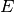, the length scale,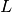, and the mass scale,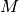. This implicitly defines the time scale,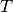, as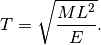The charges are typically specified in units of electron charges or in Coulombs. In PyRETIS the charges are internally scaled with the factor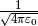(where the units of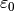is consistent with the defined,and). This means that the Coulomb constant is implicitly contained within the charges in the internal computations.

In PyRETIS, several systems of units are defined and you may also define your own units. The choice of a system of units will define the units used for input parameters. The different systems of units defined in PyRETIS are described in the following section and the creation of custom systems are also described. In the PyRETIS library, the units are defined in the pyretis.core.units module. The defined constants can be displayed by,

from pyretis.core.units import CONSTANTS
print('All constants':)
print(CONSTANTS)
print('The Boltzmann constant:')
print(CONSTANTS['kB'])


## System of units in PyRETIS¶

The systems of units that are defined in PyRETIS are:

• lj: A Lennard-Jones type of units.
• real: A system of units similar to the LAMMPS  unit real.
• metal: A system of units similar to the LAMMPS  unit metal.
• au: Atomic units. 
• electron: A system of units similar to the LAMMPS  unit electron.
• si: A system of units similar to the LAMMPS  unit si.
• gromacs: A system of units similar to the units used by GROMACS. 

The different bases for the different units are given in the table of definitions below.

The system of units is defined by setting the units keyword to any of these units, e.g.

units = real
# or perhaps:
units = metal
# or even Lennard-Jones units:
units = lj


Setting the unit system will define the units used for input to PyRETIS. The units for the different systems are given in the table of input units below. Note that the time unit is determined by the energy, length and mass scale and that the input time step will be assumed to be given in internal units. Conversion factors to more familiar units are given in the table on time units.

If the initial configuration is given as a file (for instance in xyz format), then the system of units will be used to convert the configuration. That is, PyRETIS will assume that the configuration is given in the units specified by the file format (e.g. Ångström for positions in a xyz file). The coordinates read from initial configuration fill will be converted internally to the selected system of units.

Table 45 Input units for different systems
Unit system Energy Length Mass
lj reduced reduced reduced
real kcal/mol Å g/mol
gromacs kJ/mol nm g/mol
metal eV Å g/mol
au hartree bohr electron mass
electron hartree bohr g/mol (amu)
si J m kg

For the lj system, the energy, length, mass, etc. are all given in reduced units. However, it still might be useful (for instance for trajectory output) to convert to other units. By default, the scales for the Lennard-Jones system of units corresponds to values for Argon.  These default units can be overridden explicitly by defining the length scale, mass scale and energy scale:

units = lj
units-base = {'length': (3.405, 'A'), 'mass': (39.948, 'g/mol'),
'energy': (119.8, 'kB')}


However, again note that this will only influence how we interpret input and output configurations: all other output units for this system will still be in reduced Lennard-Jones units. The syntax for overriding and creating your own units are further described in the next section.

Table 46 Defining units for energy systems
Unit system Energy unit Length unit Mass unit
lj 0.238 kcal/mol 0.3405 nm 39.948 g/mol
real 1 kcal/mol 1 Å 1 g/mol
gromacs 1 kJ/mol 1 nm 1 g/mol
metal 1 eV 1 Å 1 g/mol
au 1 hartree 1 bohr 9.10938291e-31 kg
electron 1 hartree 1 bohr 1 g/mol
si 1 J 1 m 1 kg

These units are also used for the input and define the time unit. The time units are shown in the table below. Further, all system of units expect an input temperature in Kelvin (K) and all systems, except for si, expects a charge in units of electron charges. The si system uses here Coulomb as the unit of charge. The time units for the different energy systems are given in the table below.

Table 47 Time units and velocity conversions for energy systems
Unit system Time unit
lj 2.15634977232 ps
real 48.8882129084 fs
gromacs 1.0 ps
metal 10.1805056505 fs
au 0.0241888423521 fs
electron 1.03274987345 fs
si 1.0 s

The interpretation here is that if you are for instance using the system real and would like to have a time step equal to 0.5 fs, then the input time step should be 0.5 fs / 48.8882129084 fs which is approximately 0.010227. Another example: If you are using the system metal and set your time step to 0.1, then the time step will be approximately 1.018 fs.

## Defining your own system of units¶

Defining your own system of units is basically just a matter of choosing the energy, length and mass scales. Typically, this is done by setting the units keyword and defining the base units in the section Unit-system:

System
------
units = new-system

Unit-system
-----------
name = new-system
length = (1.0, 'bohr')
mass = (9.81e-31, 'kg')
energy = (1.0, 'kcal/mol')
charge = e


Note again that this will influence how the input parameters are interpreted and that the input configuration files will be converted to the internal unit system. In the example above, a configuration read from a xyz-file will be converted from Ångström to Bohr for internal calculations. The syntax for specifying a new scale for a dimension is of form

'dimension' = (value, unit)


Where the value is a number defining the scale and unit a text string which defines the unit to use. This text string is case-sensitive. For each dimension, PyRETIS defines a set of “known” units to choose from:

Length:
• A: Ångström.
• nm: Nanometre.
• bohr: Bohr radius.
• m: Meter.
Energy:
• kcal: Kilocalorie.
• kcal/mol: Kilocalorie per mol.
• J: Joule.
• J/mol: Joule per mol.
• kJ/mol: Kilojoule per mol.
• eV: Electronvolt.
• hartree: Hartree (atomic unit of energy).
Mass:
• g/mol: Grams per mol, numerically equal to the atomic mass unit.
• g: Gram.
• kg: Kilogram.

For the charge setting, there it is currently only possible to define the unit as either e (for the electron charge) or C (for Coulomb).